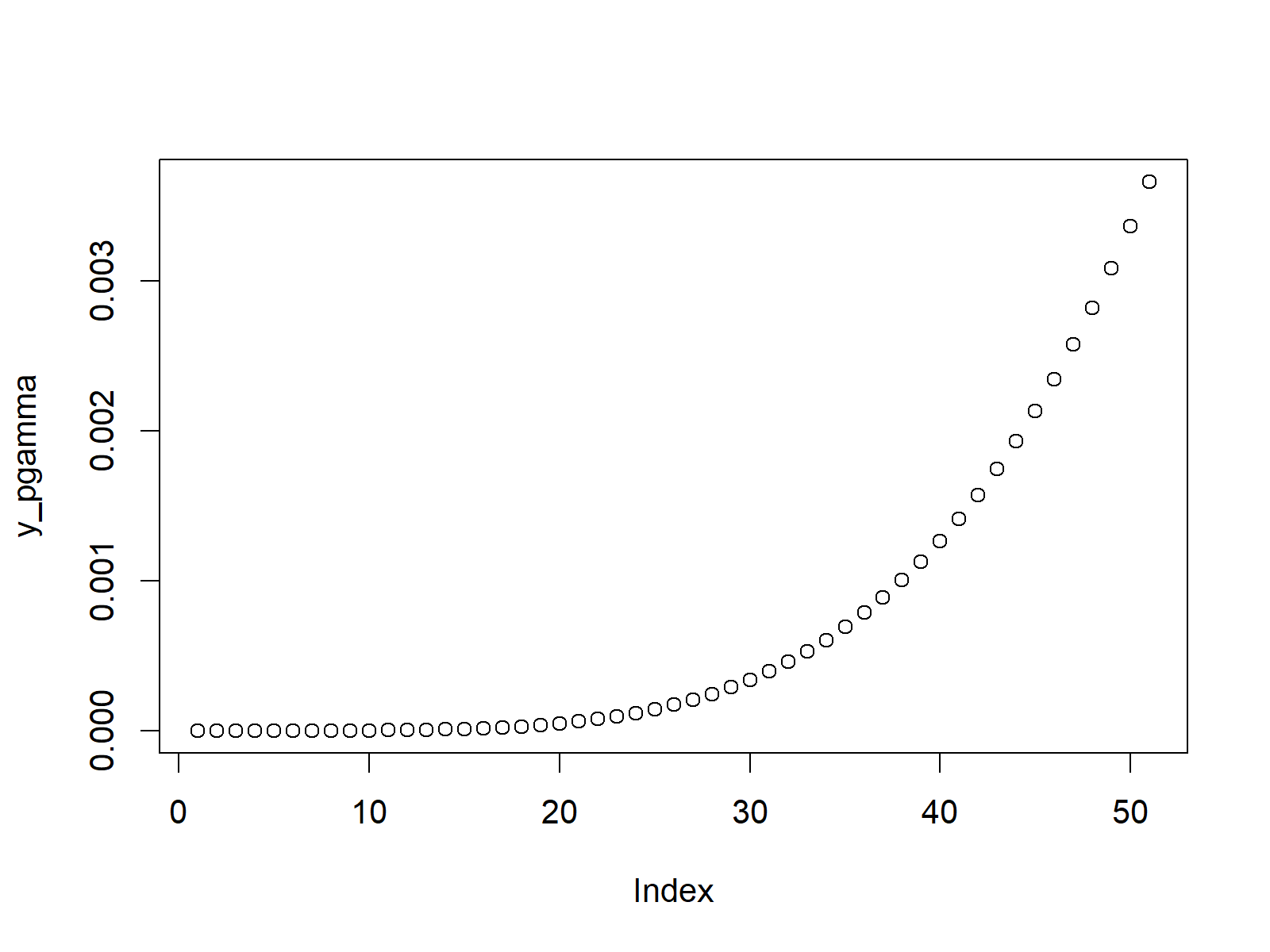# Gamma Distribution in R (4 Examples) | dgamma, pgamma, qgamma & rgamma Functions

This article illustrates how to apply the gamma functions in the R programming language.

The post is structured as follows:

Let’s dive right in:

## Example 1: Gamma Density in R (dgamma Function)

Let’s start with a density plot of the gamma distribution. For this task, we first need to create an input vector containing of a sequence of quantiles:

`x_dgamma <- seq(0, 1, by = 0.02)                     # Specify x-values for gamma function`

We can now use this vector as input for the dgamma function as you can see below. In the examples of this tutorial, we’ll use a shape of 5:

`y_dgamma <- dgamma(x_dgamma, shape = 5)              # Apply dgamma function`

In order to create a plot of the output of the dgamma function, we can apply the plot function as follows:

`plot(y_dgamma)                                       # Plot dgamma values`Figure 1: Gamma Density in R.

Figure 1 illustrates the output of the previous R syntax – A plot of the gamma distribution in R!

Let’s move on to the next example…

## Example 2: Gamma Cumulative Distribution Function (pgamma Function)

In Example 2, I’ll show you how to create a cumulative distribution function (CDF) of the gamma distribution. As in Example 1, we first need to create a sequence of quantiles:

`x_pgamma <- seq(0, 1, by = 0.02)                     # Specify x-values for pgamma function`

We can now use the pgamma function to convert our sequence of quantiles to its corresponding CDF values of the gamma distribution:

`y_pgamma <- pgamma(x_pgamma, shape = 5)              # Apply pgamma function`

We can also create a graphic of this data with the plot function in R:

`plot(y_pgamma)                                       # Plot pgamma values`Figure 2: Gamma Cumulative Distribution Function.

## Example 3: Gamma Quantile Function (qgamma Function)

If we want to create a plot reflecting the quantile function of the gamma distribution, we need to create a vector of probabilities:

`x_qgamma <- seq(0, 1, by = 0.02)                     # Specify x-values for qgamma function`

We now can use the qgamma command of the R programming language…

`y_qgamma <- qgamma(x_qgamma, shape = 5)              # Apply qgamma function`

…and create a scatterplot as follows:

`plot(y_qgamma)                                       # Plot qgamma values`Figure 3: Gamma Quantile Function.

## Example 4: Random Number Generation (rgamma Function)

In order to generate a set of random numbers, which is distributed as the gamma distribution, we need to specify a seed (for reproducibility)…

`set.seed(13579)                                      # Set seed for reproducibility`

…and we also need to specify the sample size of random numbers that we want to draw (i.e. 10000):

`N <- 10000                                           # Specify sample size`

Random numbers can now be simulated with the rgamma function:

```y_rgamma <- rgamma(N, shape = 5)                     # Draw N gamma distributed values
y_rgamma                                             # Print values to RStudio console```

Let’s have a look at a histogram of our values:

```hist(y_rgamma,                                       # Plot of randomly drawn gamma density
breaks = 1000,
main = "")```Figure 4: Random Numbers with Gamma Distribution.

Figure 4 shows the result of our random number simulation – Looks like the gamma distribution!

## Video & Further Resources

Have a look at the following video of my YouTube channel. I’m explaining the content of this post in the video:

You might also have a look at the other tutorials on distributions and the simulation of random numbers in the R programming language:

Furthermore, you could read the other posts on this homepage. You can find some tutorials below:

Summary: You learned in this tutorial how to use the different gamma functions in R. Please let me know in the comments, if you have additional questions or comments.

Subscribe to the Statistics Globe Newsletter